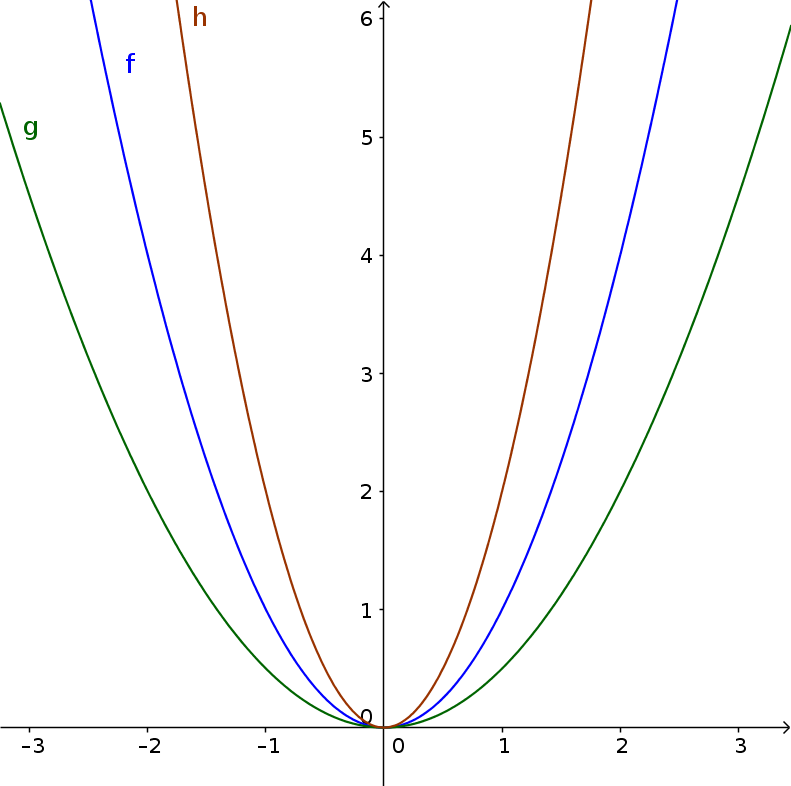Math Quadratic functions Stretching and compressing the standard parabola

# Stretching and compressing the standard parabola

The standard parabola can be stretched and compressed with the parameter $a$. The general formula is:

$y=ax^2$
!

### Remember

• If $|a|$ > 1, the graph is steeper than the standard parabola and is stretched.
• If $|a|$ < 1, the graph is flatter than the standard parabola and is compressed.

### Example

$\color{green}{g(x)=\frac12x^2}$
$\color{blue}{f(x)=x^2}$
$\color{brown}{h(x)=2x^2}$